# In each part, solve the linear system, if possible, and use the result to determine whether...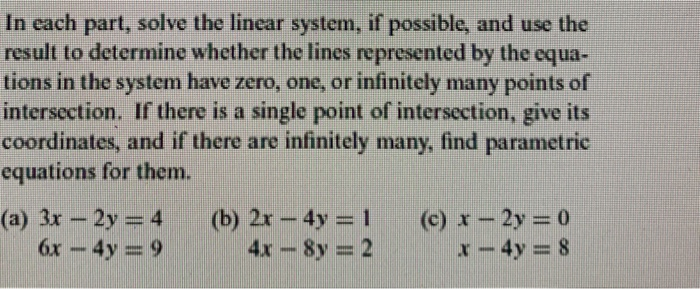In each part, solve the linear system, if possible, and use the result to determine whether the lines represented by the equa- tions in the system have zero, one, or infinitely many points of intersection. If there is a single point of intersection, give its coordinates, and if there are infinitely many, find parametric equations for them. (a) 3x – 2y = 4 (b) 2x - 4y = 1 (c) x - 2y = 0 6x – 4y = 9 4x - 8y = 2 x - 4y = 8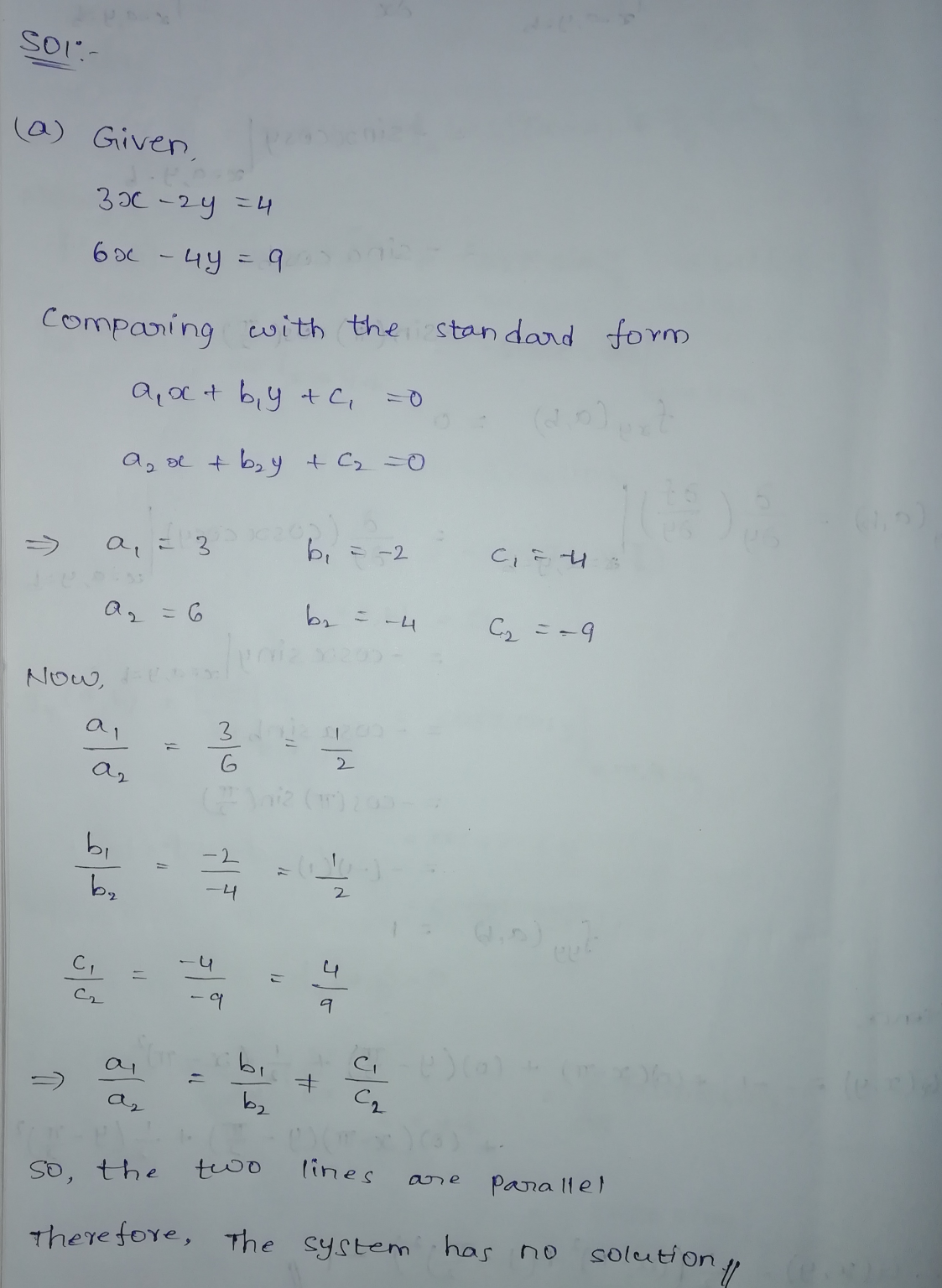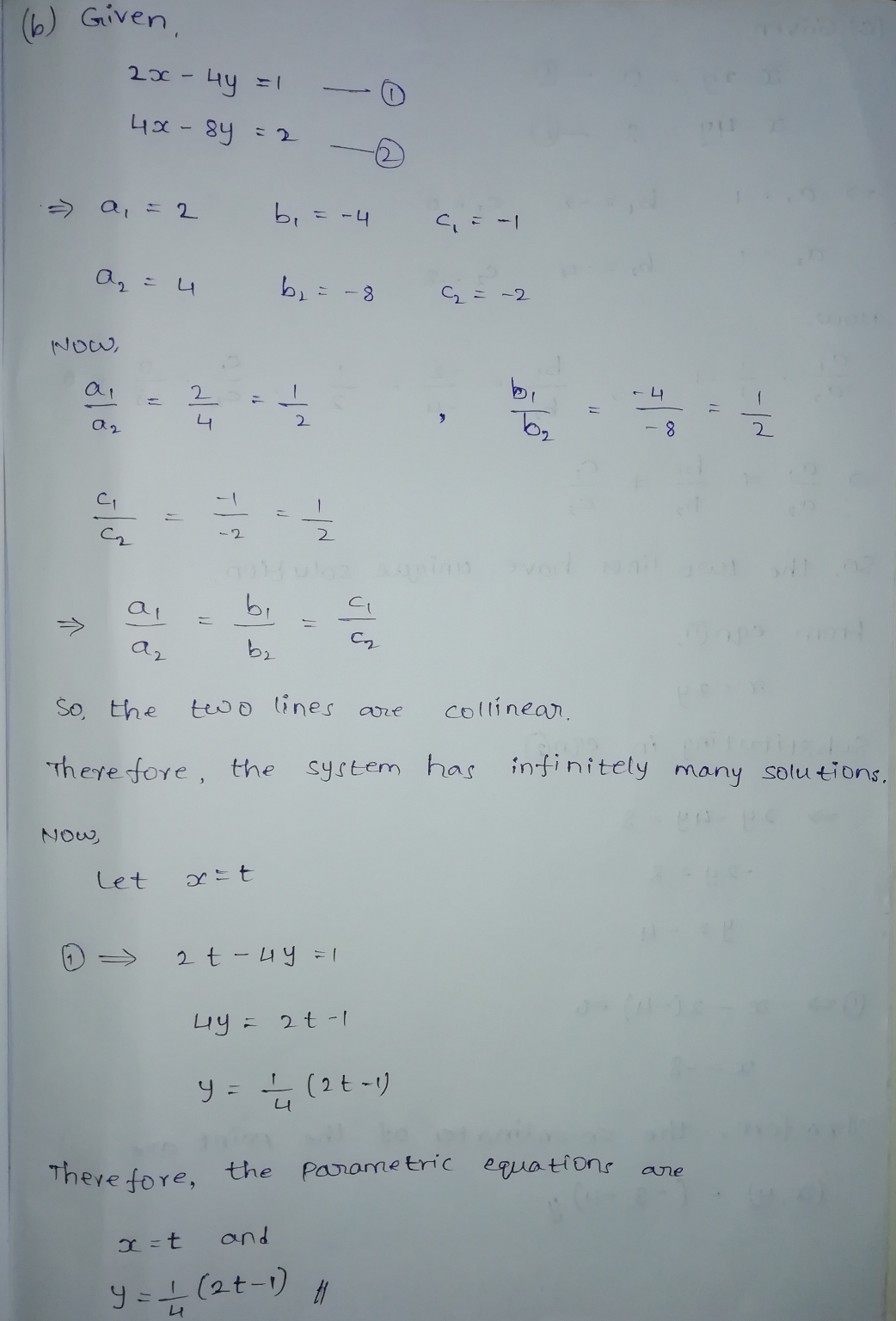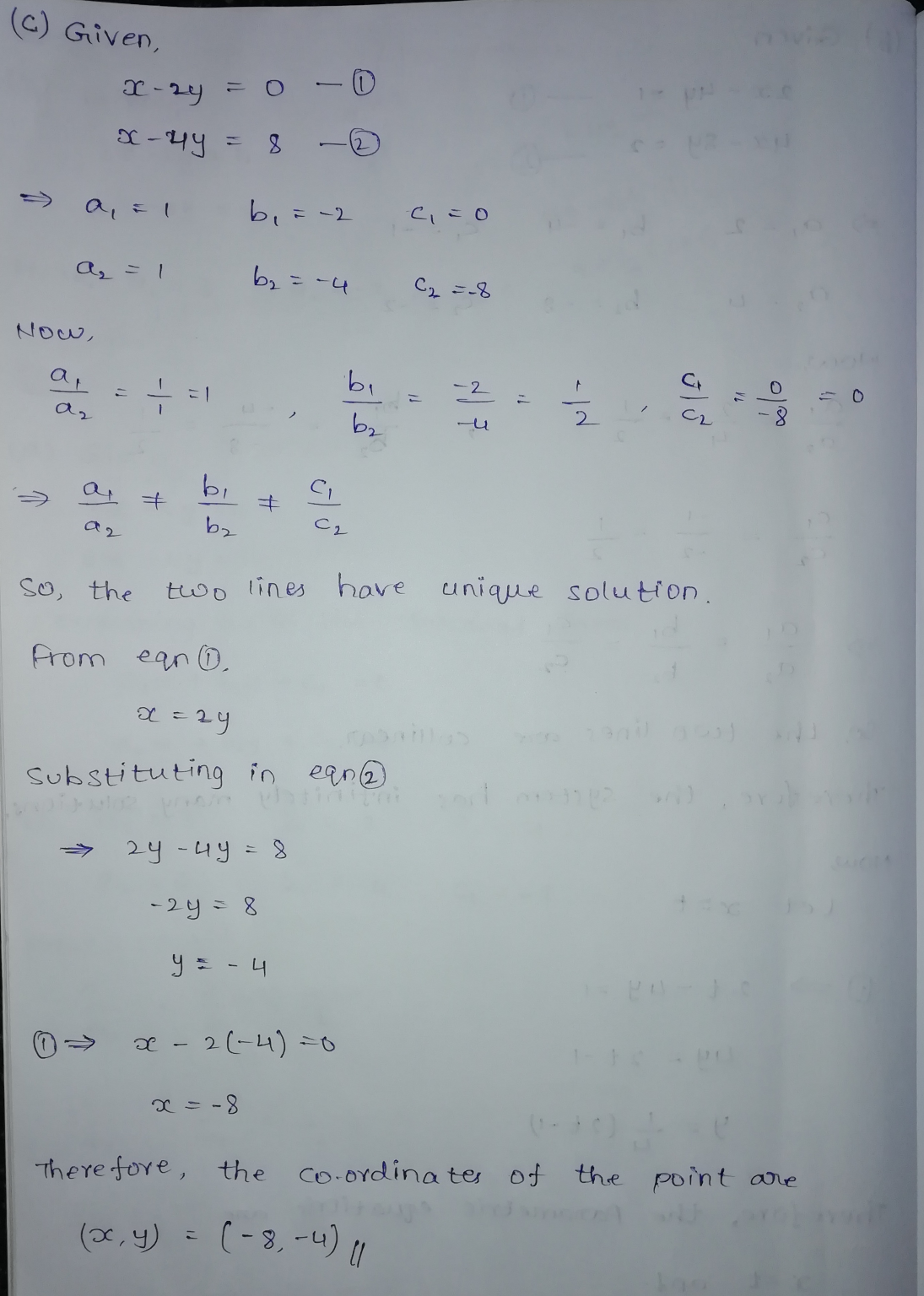If you have any dought please comment and give me a like if the answer is clear. Thank you

##### Add Answer of: In each part, solve the linear system, if possible, and use the result to determine whether...
Similar Homework Help Questions
• ### Determine whether each system of linear equations has a)one and only one solution, b)infinitely many solutions, or c) no solution 2x-4y=5 3x +2y=6

Determine whether each system of linear equations has a)one and only one solution, b)infinitely many solutions, or c) no solution2x-4y=53x +2y=6

• ### Solve the following system of equations. (Enter your answers as a comma-separated list. If there are...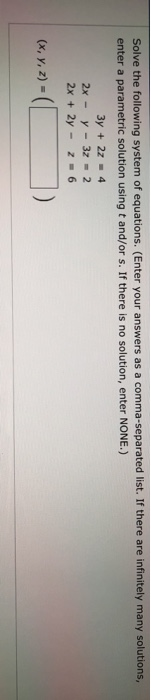Solve the following system of equations. (Enter your answers as a comma-separated list. If there are infinitely many solutions, enter a parametric solution using t and/or s. If there is no solution, enter NONE.) 3y + 2z 4 2x-y-3z 2 2x 2y z6 (x, y, 2) Solve the following system of equations. (Enter your answers as a comma-separated list. If there are infinitely many solutions, enter a parametric solution using t and/or s. If there is no solution, enter NONE.)...

• ### i need help on these 4: *4y=15-3x 2y=3x+21 *4x=5y-14 y=-6x-9 *5x=4y-30 2x=3y=-12 *2/3y=10+4x 5x=1/3y-8 use linear combinations to solve the system of linear equations thank you

PLEASE!!!!i need help on these 4:*4y=15-3x2y=3x+21*4x=5y-14y=-6x-9*5x=4y-302x=3y=-12*2/3y=10+4x5x=1/3y-8use linear combinations to solve the system of linear equationsthank you!!!

• ### Determine whether the guiven order pair is a solution of the system of equations 1

Determine whether the guiven order pair is a solution of the system of equations 1. (1,-1) 3x-2y=5 -2x+4y=6 2. (-2,3) x-y = -5 -3x+2y=12 Solve Graphically x+y=2 2x-y=1 2x+y=0 3x-5y=0 4x-2y=10 x+3y=6

• ### Solve the system of linear equations, using the Gauss-Jordan elimination method

Solve the system of linear equations, using the Gauss-Jordan elimination method. (If there is no solution, enter NO SOLUTION. If there are infinitely many solutions involving one parameter, enter the solution using t for the last variable. If the solution involves two parameters, add s for the second to last variable.)4x−8y−24z=−20x−2y−6z=−53x−6y−18z=15

• ### Name Date Period Kuta Software Solving Systems of Equations by Substitution Solve each system by substitution....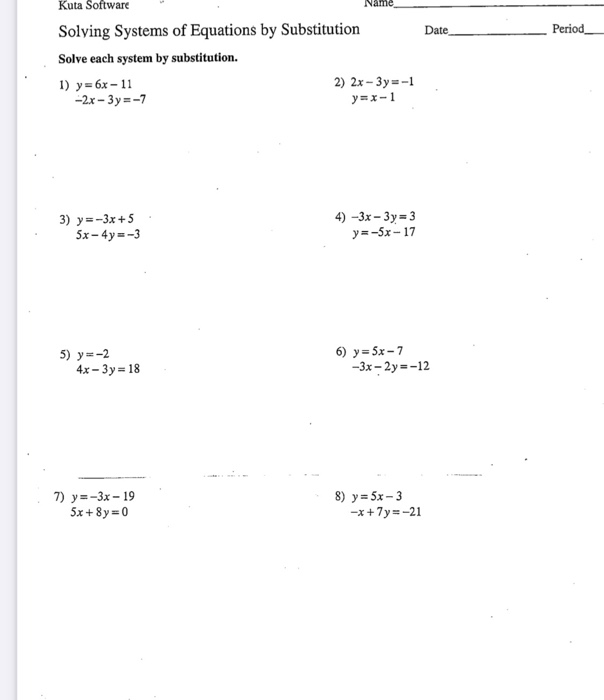Name Date Period Kuta Software Solving Systems of Equations by Substitution Solve each system by substitution. 1) y=6x-11 2) 2x - 3y = -1 -2x - 3y =-7 y=x-1 3) y=-3x + 5 5x - 4y=-3 4) -3x – 3y = 3 y=-5x-17 5) y=-2 4x - 3y = 18 6) y = 5x - 7 -3x - 2y=-12 7) y=-3x - 19 5x + 8y = 0 8) y = 5x - 3 -x + 7y=-21

• ### Hello out there,I need some help with some problems,the first one is,Solve the system of linear equations by graphing:4x-y=6 and 3x+y=1

Hello out there,I need some help with some problems,the first one is,Solve the system of linear equations by graphing:4x-y=6 and 3x+y=1.The next one is,Determine whether the system has one solution,no solution,or infinitely many solutions:y=-x+2 and 3x+3y=6.The third one is,Determine whether the system has one solution,no solution,or infinitely many solutions:y=2/3x and 2x-y=-4.And the last one is,Solve the system of equations by substitution:y=x^2+2x-2 and y=3x+4.Thanks to whoever can help.

• ### Solve each system of equations, give the solution and state whether there are no solutions or many solutions

Solve each system of equations, give the solution and state whether there are no solutions or many solutions. 1 )3x + 8y = 1 7x + 4y = 17 Answer: (3,1) 2) -3x + 5y = -7 9x + 2y = 38 Answer: (4,1) 3) 7r + 3s = 13 14r - 15s = -16 Answer: (1,2) 4) 2x - 7y = 42 4x - 14y = 64 Answer: No Solution ?? 5) 9x + 12y = 15 3x +...

• ### Hello out there,I really need some help with some tough problems,the first one is Solve the system of linear equations by graphing:4x-y=6 and 3x+y=1

Hello out there,I really need some help with some tough problems,the first one is Solve the system of linear equations by graphing:4x-y=6 and 3x+y=1.The next one is ,State whether the system is consistent and independent,consistent and dependent,or inconsistent:4x+2y=8 and 2x+y=1.The third one is,State whether the system is consistent and independent,consistent and dependent,or inconsistent:x+y=4 and 2x-y=-7.And the last one is,Determine whether the system has one solution,no solution,or infinitely many solutions,y=-x+2 and 3x+3y=6.Thanks to whoever can help me.

• ### Linear Equations

Determine whether each system of linear equations has one and only one solution, infinitely many solutions, or no solution. Find all solutions whenever theyexist.1) x + 2y = 72x – y = 52) 2x – 5y = 106x – 15y = 30

Free Homework App